# Branchless Matrix to Quaternion Conversion

(Edit: This arti­cle is a more in-depth write­up of an algo­rithm that I devel­oped around 2005, and first post­ed to Mar­tin Baker’s Euclid­ean Space web­site. That time was the height of the Intel Net­Burst archi­tec­ture, which was noto­ri­ous for its deep pipeline and high branch mis­pre­dic­tion penal­ty. Hence the moti­va­tion to devel­op a branch-free matrix to quater­nion con­ver­sion rou­tine. What fol­lows is the com­plete deriva­tion and analy­sis of this idea.)

The orig­i­nal rou­tine to con­vert a matrix to a quater­nion was giv­en by Ken Shoe­make  and is very branchy. There is a way to elim­i­nate these branch­es and arrive at a com­plete­ly branch-free and high­ly par­al­leliz­able code. The trade off is the intro­duc­tion of 3 addi­tion­al square roots. Jump to the analy­sis sec­tion and the end of this arti­cle, or con­tin­ue fist with the math bits.

## Trade 3 roots for a branch

When a quater­nion with ele­ments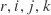is con­vert­ed to a matrix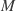, the terms that final­ly end up in the matrix can be added and sub­tract­ed to arrive at a wealth of iden­ti­ties. The ele­ments in the matrix diag­o­nal are relat­ed to the quater­nion ele­ments by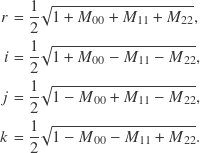The off-diag­o­nal matrix ele­ments are relat­ed to the quater­nion ele­ments by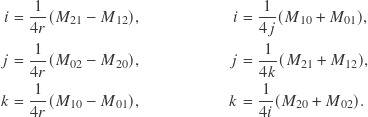It is obvi­ous that there are mul­ti­ple paths to restore the quater­nion from a matrix. One could first restorefrom the diag­o­nal ele­ments, and then use the off-diag­o­nal ele­ments to restore,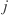and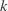. Or first restorefrom the diag­o­nal ele­ments, and then use the off-diag­o­nal for the rest, etc. The stan­dard, branchy con­ver­sion code just does that. Can we elim­i­nate the branch­es and just use the diag­o­nal ele­ments for every­thing? In the­o­ry, yes, the only thing miss­ing would be the signs.

void BranchlessMatrixToQuaternion( float out, float in ) { out = .5 * sqrt( max( 0, 1 + in + in + in ) ); // r out = .5 * sqrt( max( 0, 1 + in - in - in ) ); // i out = .5 * sqrt( max( 0, 1 - in + in - in ) ); // j out = .5 * sqrt( max( 0, 1 - in - in + in ) ); // k out = copysign( out, in - in ); // i out = copysign( out, in - in ); // j out = copysign( out, in - in ); // k }

Math­e­mat­i­cal­ly, if the matrixhas a deter­mi­nant of one, then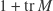can­not be neg­a­tive, and all four square roots are defined. In prac­tice there can be round­ing errors, so to be safe the terms are clamped with max( 0, ... ) before the square roots are tak­en. The off diag­o­nal ele­ments are only used to restore the miss­ing signs. Since only the signs mat­ter, the divi­sion bycan be lift­ed! (The func­tion copysign is sup­posed to trans­fer the sign bit from one float­ing point num­ber to anoth­er, and your com­pil­er should do a decent job and reduce it to some sequence of bit­wise and/or instruc­tions. Clang and GCC do this on x86 with SSE float­ing point. See the com­ment sec­tion for a dis­cus­sion about this.)

Com­pared with the orig­i­nal con­ver­sion code, there are four square roots instead of one square root and one divi­sion, but there aren’t any branch­es. The code pipelines well (all the square roots can run in par­al­lel, fol­lowed by all the copysigns).

## Operation Counts

In the fol­low­ing table of oper­a­tion counts, I have includ­ed a max( 0, ... ) safe­guard also in the Shoe­make algo­rithm (the orig­i­nal does­n’t have this). The copysign is regard­ed as a sin­gle operation.

Shoe­make Branch­less
div/sqrt 2 4
float­ing point
compare
1..3 0
branch 1..3 0
copy­sign 0 3
steps in longest
depen­den­cy chain
8..15 6

Let’s assume the laten­cy of sqrt and div as 15 cycles (sin­gle pre­ci­sion), the laten­cy of a branch as 2 cycles with a mis­pre­dic­tion penal­ty of 15 cycles, and the aver­age laten­cy of every­thing else as 3 cycles. These num­bers are in the ball­park of Intel Wolf­dale resp. AMD Jaguar proces­sors . Depend­ing on whether the exe­cu­tion is assumed to be either per­fect­ly ser­i­al and in-order (a sim­ple addi­tion of all the laten­cies), or assumed to be per­fect­ly par­al­lel and out-of-order (the sum of the laten­cies in the longest depen­den­cy chain), we get the cycle counts shown in the table below. The real­i­ty should be any­where between these extremes.

Shoe­make Branch­less
per­fect­ly ser­i­al (in-order) 68..129 138
per­fect­ly par­al­lel (out of order) 49..88 30

## Numerical Stability

The catch of the branch­less algo­rithm is that the sin­gu­lar­i­ty at the rota­tion angle of 180° is still there. Although the divi­sion bywas lift­ed and so a divi­sion by zero can­not occur, it now appears as ran­dom signs from cat­a­stroph­ic can­cel­la­tion. Take for exam­ple, a 180° rota­tion around the x‑y diagonal,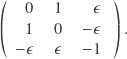In an ide­al world, when the rota­tion angle trav­els through a small neigh­bor­hood around the point of 180°, the val­ue of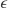van­ish­es and changes sign. Theandcom­po­nents of the result­ing quater­nion should then flip signs simul­ta­ne­ous­ly too. In real­i­ty how­ev­er, with just the tini­est amount of round­ing error, these signs are qua­si ran­dom. The behav­ior can be described like this:

just before 180°
(con­sis­tent signs)
at 180°
(ran­dom signs)
just after 180°
(con­sis­tent signs)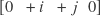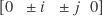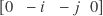## Recommendation

A con­fig­u­ra­tion which pro­vokes the sin­gu­lar­i­ty described above is sur­pris­ing­ly com­mon as an exchange of coor­di­nate axes (con­ver­sion from Z‑up to Y‑up, for exam­ple). Where the branch­less algo­rithm shines how­ev­er is the con­ver­sion of local ani­ma­tion keys. These rarely cross a 180° rota­tion angle. In one use case for me, the skele­ton is the skele­ton of a plant and the ani­ma­tion is dri­ven by physics, and I know or can make sure that a rota­tion of 180° sim­ply does­n’t occur.

Giv­en this knowl­edge, the branch­less con­ver­sion code is rec­om­mend­ed over the stan­dard one, under the con­di­tion that the rota­tion angle does not cross 180°.

## References

 Ken Shoe­make, “Quater­nions”,
http://www.cs.ucr.edu/~vbz/resources/quatut.pdf

 Instruc­tion Tables at Agn­er Optimization
http://www.agner.org/optimize/instruction_tables.pdf

## 4 Gedanken zu „Branchless Matrix to Quaternion Conversion“

1. On PPC archi­tec­tures, I would rec­om­mend *not* doing copy­sign() with bit oper­a­tions, because that incurs a LHS penal­ty. On those archi­tec­tures, you are bet­ter of imple­ment­ing copy­sign() using fabs() and a float­ing-point select.

Like the branch­less ver­sion, will come in handy in the future!

2. Dear Chris­t­ian,
See below f90 implementation.
Alex
===========================================

function mtrx2quat1(r)
! get rotation quaternion from matrix
! Method: http://www.thetenthplanet.de/archives/1994
! tested OK
type(TQUAT)          :: mtrx2quat1
real(SP),intent(IN) :: r(3,3)

real(SP) :: s,t,sq2=sqrt(0.5_SP)

t=(r(1,1)+r(2,2)+r(3,3))*0.5_SP
s=0.5_SP-t
mtrx2quat1%q(0)=sq2*sqrt(max(0._SP,0.5+t))
mtrx2quat1%q(1)=sign(sq2*sqrt(max(0._SP,r(1,1)+s)),r(3,2)-r(2,3))
mtrx2quat1%q(2)=sign(sq2*sqrt(max(0._SP,r(2,2)+s)),r(1,3)-r(3,1))
mtrx2quat1%q(3)=sign(sq2*sqrt(max(0._SP,r(3,3)+s)),r(2,1)-r(1,2))
end function mtrx2quat1

• Hi Alex,
Yes, the code that I showed in the arti­cle does not aggres­sive­ly com­bine com­mon subex­pres­sions, as your code does. I did this for clar­i­ty and under the assump­tion that the com­pil­er could do some­thing sim­i­lar, if giv­en the lib­er­ty to do so (eg. ‑ffast-math and co). In any case, thanks for your contribution!

EDIT: On a side note, fac­tor­ing out com­mon sub expres­sions increas­es the length of the depen­den­cy chain, so it would be arguable if that is real­ly faster with out-of-order exe­cu­tion. In any case, mea­sure­ment over opinion!